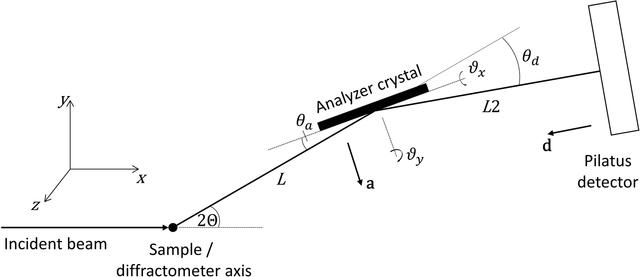disable zoom     view article Figure 2 Schematic illustration of the mechanical setup with a single analyzer crystal. 2Θ is the angle of the diffractometer arm, θa the Bragg angle of the analyzer crystal, θd the rotation of the detector about the analyzer axis (typically twice θa), a a unit vector normal to the analyzer and d a unit vector normal to the detector. L and L2 are the nominal distances between the diffractometer axis and the analyzer crystal, and between the analyzer crystal and the detector, respectively. With 2Θ = θa = 0, ϑx is the rotation of the analyzer crystal about the x direction (roll aberration) and ϑy the subsequent rotation about the y direction (yaw aberration). If ϑx is zero, ϑy corresponds to a rotation about the crystal normal, so has no effect on diffraction.JOURNAL OFAPPLIEDCRYSTALLOGRAPHY
ISSN: 1600-5767
Volume 54| Part 4| August 2021| Pages 1088-1099